# Approximate Square Roots and Irrational Numbers Lesson Plan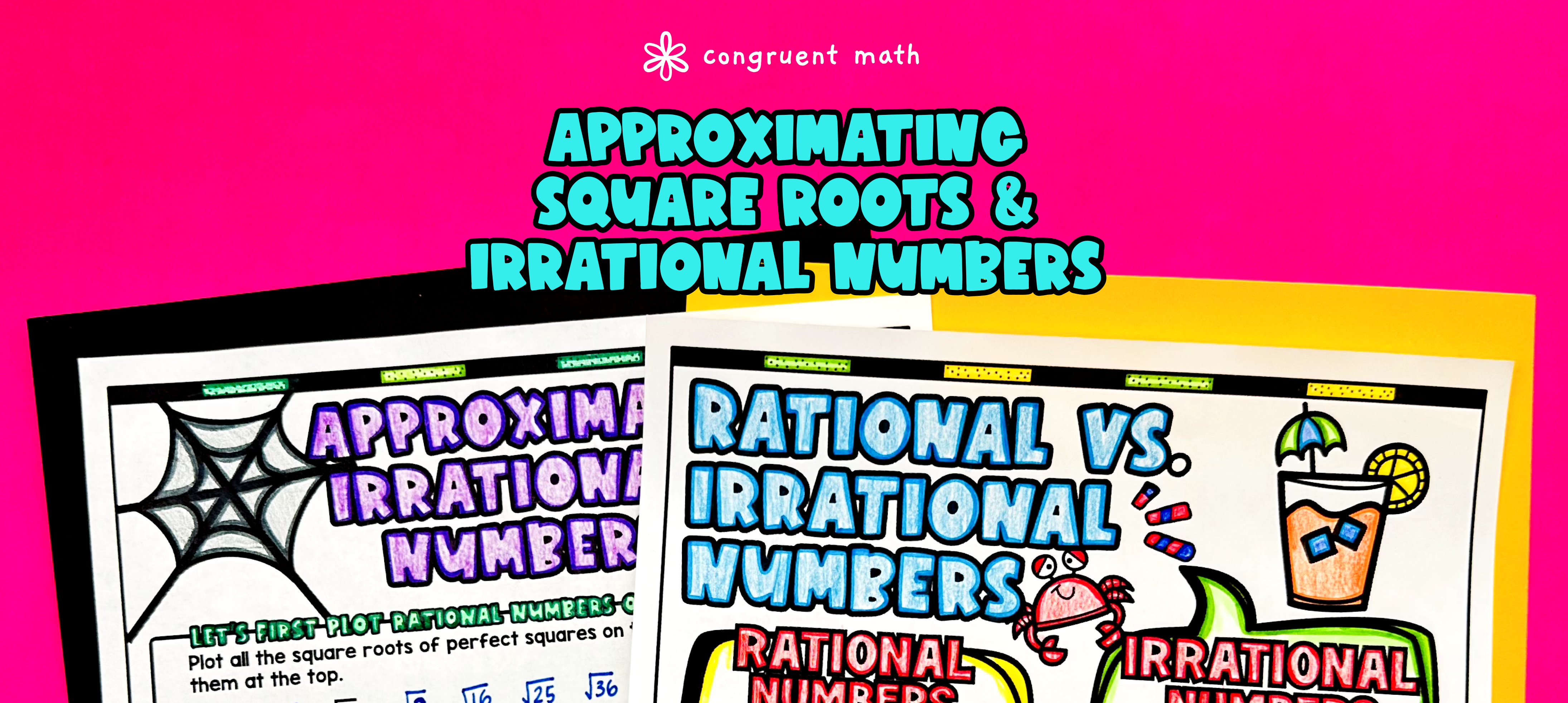Pin This

## Overview

Ever wondered how to teach approximate square roots and irrational numbers in an engaging way to your eighth-grade students?

In this lesson plan, students will learn about the concept of square roots and their real-life applications. Through artistic, interactive guided notes, checks for understanding, practice worksheets, and a color by number activity, students will gain a comprehensive understanding of approximate square roots and locating irrational numbers on the number line.

The lesson culminates with a real-life example that explores how these concepts can be applied to approximate pi and use it in calculations involving circles. This lesson is perfect for introducing the topic, reviewing or reteaching, homework assignments, sub plans, or quiz/test preparations. With its engaging activities and real-life connections, students will have an enjoyable and meaningful learning experience.

## Get the Lesson Materials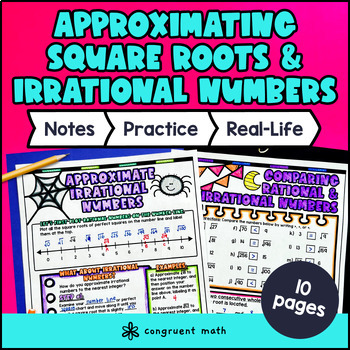\$4.25

## Learning Objectives

After this lesson, students will be able to:

• Approximate square roots of non-perfect squares

• Locate irrational numbers on a number line

• Compare and order irrational numbers

• Explain the concept of irrational numbers and their real-life applications

## Prerequisites

Before this lesson, students should be familiar with:

• Basic multiplication and division skills

• Understanding of the concept of square roots

• Basic understanding of rational numbers and their properties

• Understanding of the number line and how to plot points on it

## Key Vocabulary

• Approximate square roots

• Irrational numbers

• Number lines

## Procedure

### Introduction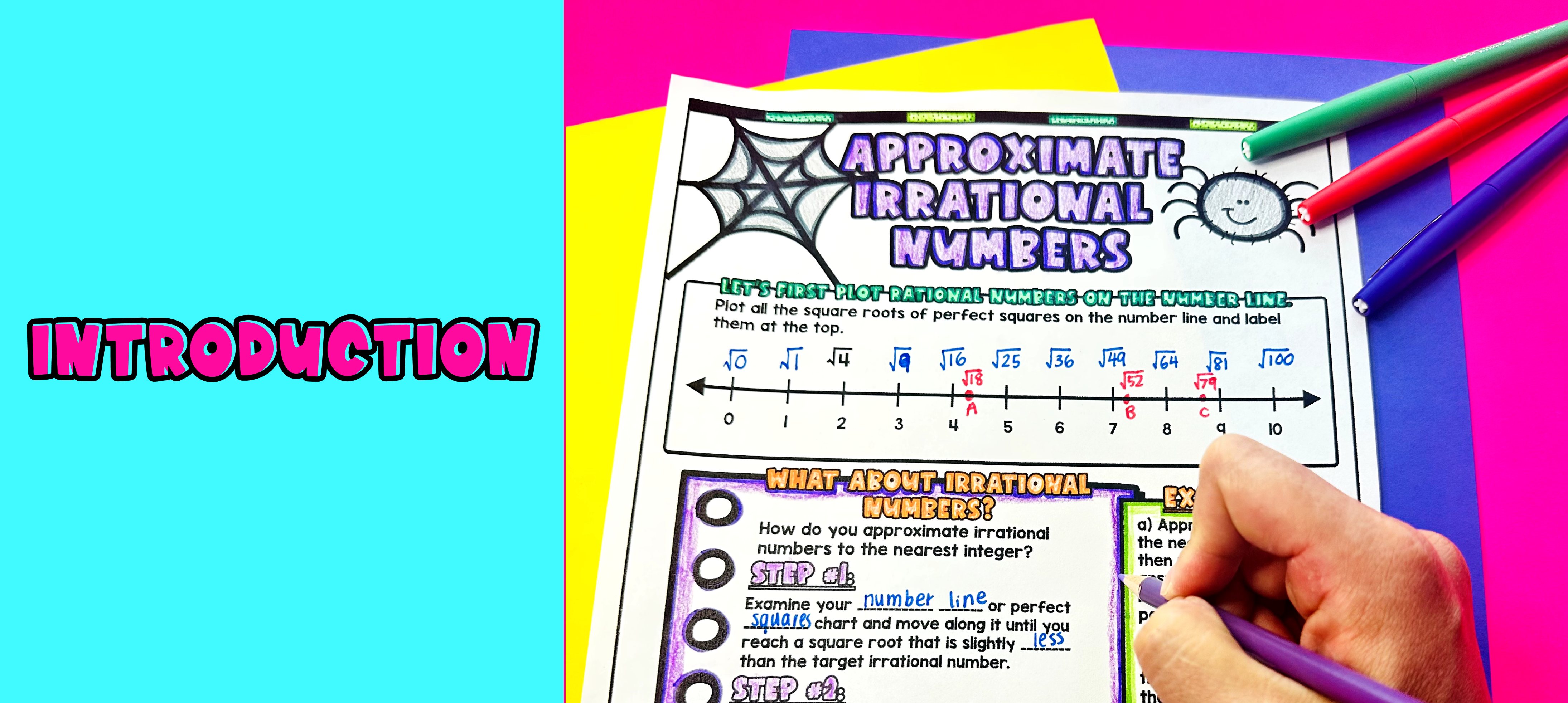Pin This

As a hook, ask students why it is important to approximate square roots and locate irrational numbers on a number line. Refer to the last page of the guided notes as well as the FAQs below for ideas.

Use the guided notes to introduce the concept of approximating square roots and locating irrational numbers on a number line. Walk through the key points of the topic, emphasizing the importance of understanding irrational numbers and their approximate values. Discuss the process of approximating square roots by finding perfect squares and using number lines. Refer to the FAQ below for a walk through on this, as well as ideas on how to respond to common student questions.

• Check for Understanding: Have students walk through the examples of comparing and finding consecutive numbers after a square root on the second page of the guided notes. Call on students to talk through their answers, potentially on the whiteboard or projector. Based on student responses, reteach concepts that students need extra help with.

Based on student responses, reteach concepts that students need extra help with. If your class has a wide range of proficiency levels, you can pull out students for reteaching, and have more advanced students begin work on the practice exercises.

### Practice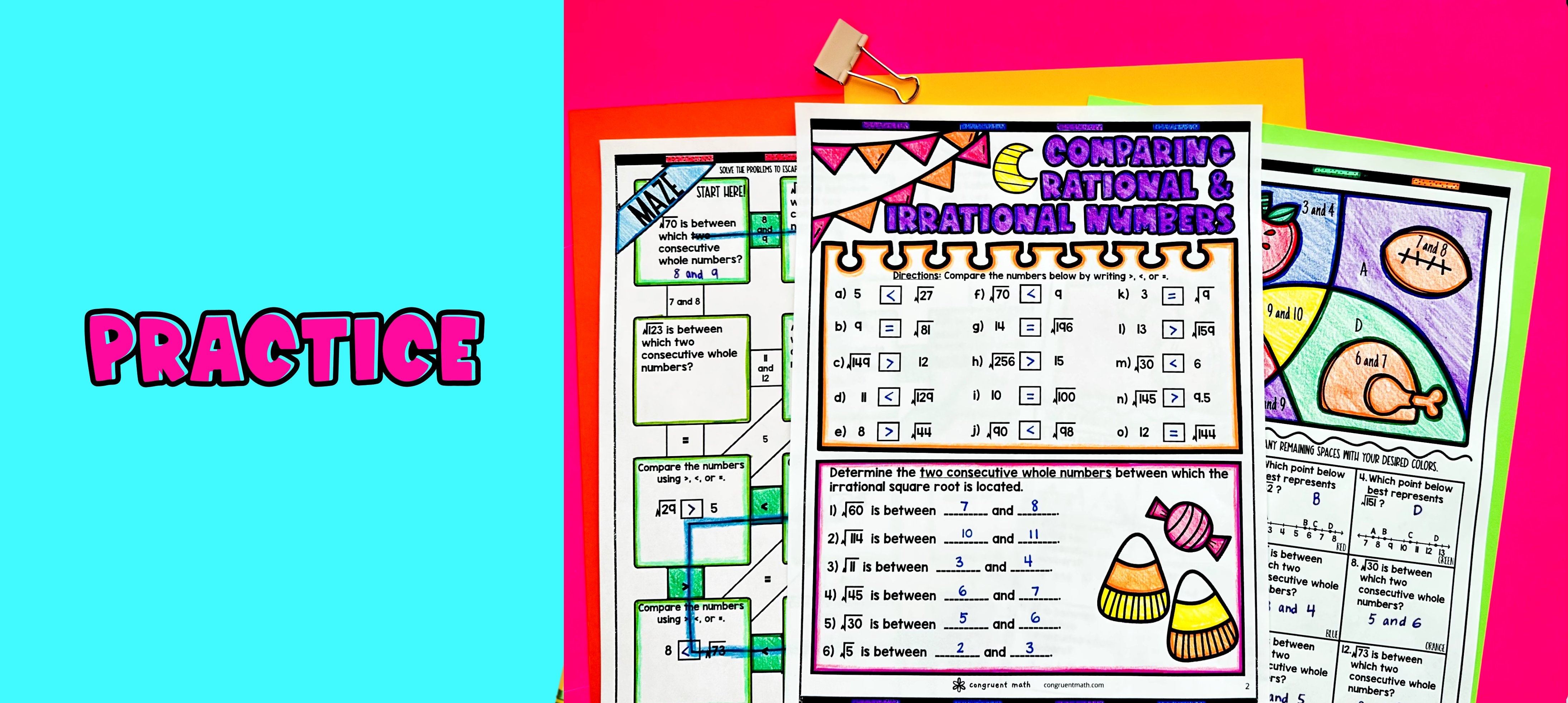Pin This

Have students practice approximating square roots and locating irrational numbers on the number line using the practice maze activity provided. Walk around the classroom to answer any student questions and provide guidance as needed.

Fast finishers can dive into the color by code activity for extra practice. You can assign this activity as homework for the remainder of the class.

### Real-Life Application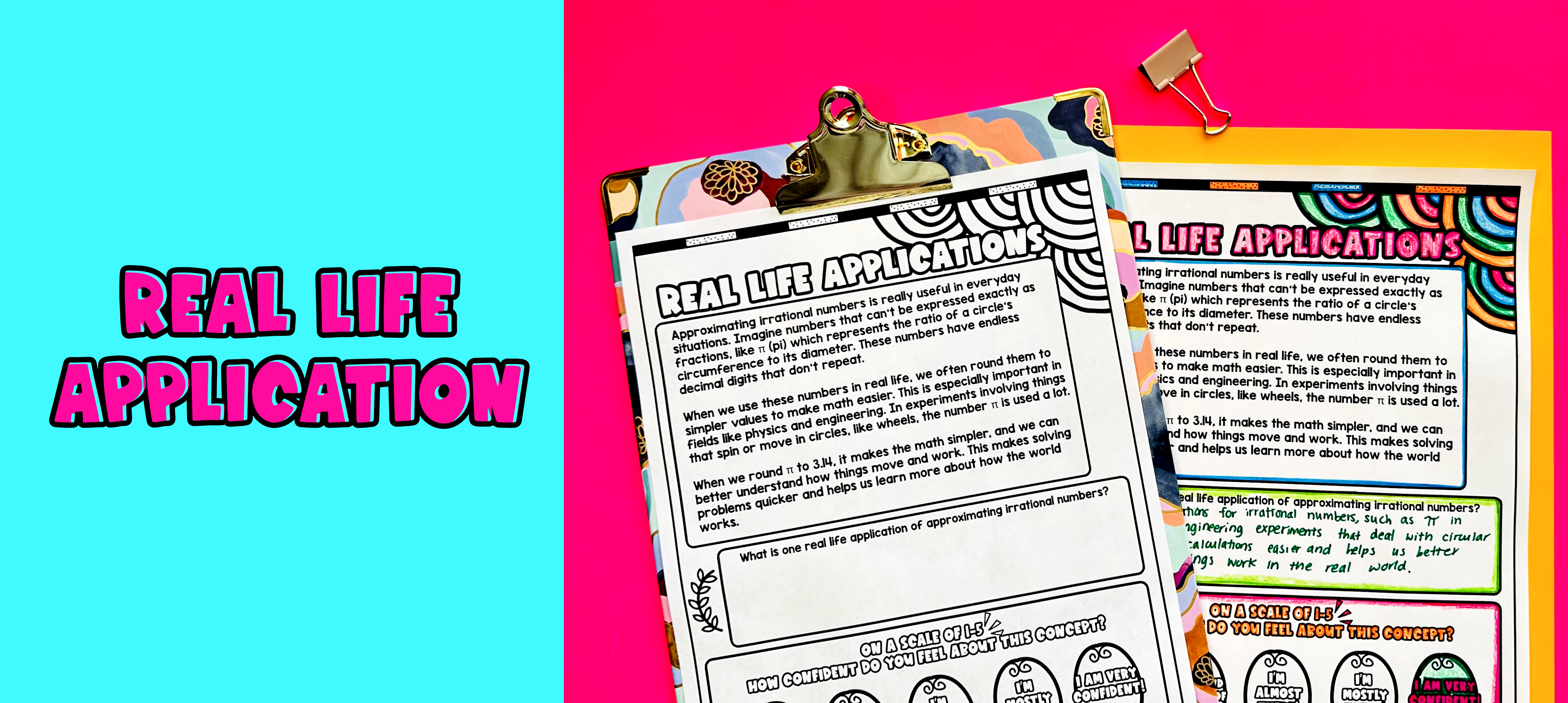Pin This

Bring the class back together, and introduce the concept of using approximate square roots and irrational numbers to approximate the value of pi and use it for calculations involving circles. Refer to the FAQ for more ideas on how to teach it!